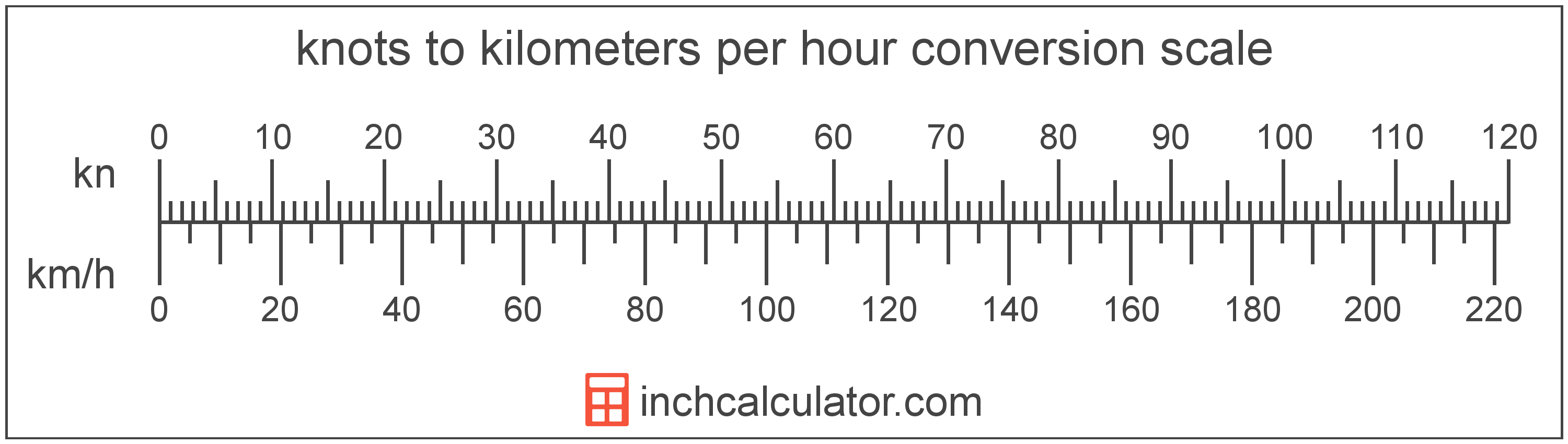# Knots to Kilometers Per Hour Conversion

Enter the speed in knots below to get the value converted to kilometers per hour.

Results in Kilometers Per Hour:1 kn = 1.852 km/h

## How to Convert Knots to Kilometers Per HourTo convert a knot measurement to a kilometer per hour measurement, multiply the speed by the conversion ratio. One knot is equal to 1.852 kilometers per hour, so use this simple formula to convert:

kilometers per hour = knots × 1.852

The speed in kilometers per hour is equal to the knots multiplied by 1.852.

For example, here's how to convert 5 knots to kilometers per hour using the formula above.
5 kn = (5 × 1.852) = 9.26 km/h

## Knots

One knot is equal to a speed of one nautical mile per hour, or one minute of latitude per hour.

Knots can be abbreviated as kn, and are also sometimes abbreviated as kt. For example, 1 knot can be written as 1 kn or 1 kt.

## Kilometers Per Hour

Kilometers per hour are a measurement of speed expressing the distance travelled in kilometers in one hour.

The kilometer per hour, or kilometre per hour, is an SI unit of speed in the metric system. Kilometers per hour can be abbreviated as km/h, and are also sometimes abbreviated as kph. For example, 1 kilometer per hour can be written as 1 km/h or 1 kph.

Kilometers per hour can be expressed using the formula:
vkm/s = dkmthr

The velocity in kilometers per hour is equal to the distance in kilometers divided by time in hours.

## Knot to Kilometer Per Hour Conversion Table

Knot measurements converted to kilometers per hour
Knots Kilometers Per Hour
1 kn 1.852 km/h
2 kn 3.704 km/h
3 kn 5.556 km/h
4 kn 7.408 km/h
5 kn 9.26 km/h
6 kn 11.11 km/h
7 kn 12.96 km/h
8 kn 14.82 km/h
9 kn 16.67 km/h
10 kn 18.52 km/h
11 kn 20.37 km/h
12 kn 22.22 km/h
13 kn 24.08 km/h
14 kn 25.93 km/h
15 kn 27.78 km/h
16 kn 29.63 km/h
17 kn 31.48 km/h
18 kn 33.34 km/h
19 kn 35.19 km/h
20 kn 37.04 km/h
21 kn 38.89 km/h
22 kn 40.74 km/h
23 kn 42.6 km/h
24 kn 44.45 km/h
25 kn 46.3 km/h
26 kn 48.15 km/h
27 kn 50 km/h
28 kn 51.86 km/h
29 kn 53.71 km/h
30 kn 55.56 km/h
31 kn 57.41 km/h
32 kn 59.26 km/h
33 kn 61.12 km/h
34 kn 62.97 km/h
35 kn 64.82 km/h
36 kn 66.67 km/h
37 kn 68.52 km/h
38 kn 70.38 km/h
39 kn 72.23 km/h
40 kn 74.08 km/h

## References

1. NASA, Knots Versus Miles per Hour, https://www.grc.nasa.gov/WWW/K-12/WindTunnel/Activities/knots_vs_mph.html